# 一种基于地图匹配和变化区域监测的路网核查方法A Method of Road Network Verification Based on Map Matching and Change Area Monitoring

DOI: 10.12677/GST.2020.83012, PDF, HTML, XML, 下载: 23  浏览: 64  国家自然科学基金支持

Abstract: Road network verification refers to the use of various technical means to verify the authenticity of road network data reported by different regions. The traditional verification methods include manual field inspection, manual field comparison and other methods, with low efficiency. In this paper, a “full-automatic screening + suspected manual review” method is used to improve the effi-ciency of road network verification, and a quantitative reference evaluation index is proposed to eliminate the difference of verification results caused by the different evaluation standards of dif-ferent operators. It is a convenient solution to utilize the high-speed development of space-time trajectory of floating car and high-resolution remote sensing technology.

1. 引言

2. 相似性特征设计与表达

a) 基于面积累积的形状差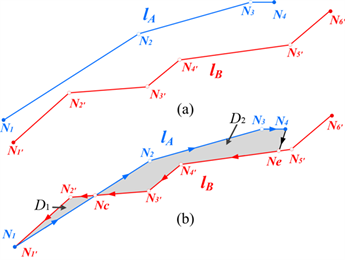Figure 1. Schematic diagram of shape difference between road pairs to be matched

${l}_{A}$${l}_{B}$ 基于面积累积的形状差即为封闭区域的多边形面积积分，如下：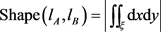(1)

${\iint }_{D}\left(\frac{\partial Q}{\partial x}-\frac{\partial P}{\partial y}\right)\text{d}x\text{d}y={\oint }_{L}P\text{d}x+Q\text{d}y$ (2)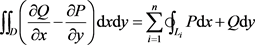(3)

$y={k}_{i}x+{b}_{i}$ (4)

$-{\iint }_{D}\text{d}x\text{d}y=\underset{i=1}{\overset{n}{\sum }}{\oint }_{{L}_{i}}\left({k}_{i}x+{b}_{i}\right)\text{d}x$ (5)

$\text{Shape}\left({l}_{A},{l}_{B}\right)=|-{\int }_{{x}_{i}}^{{x}_{i+1}}\left({k}_{i}x+{b}_{i}\right)\text{d}x|$ (6)

b) 长度相似性特征

$\text{simLen}\left({l}_{A},{l}_{B}\right)=1-\frac{|\mathrm{Len}\left({l}_{A}\right)-\mathrm{Len}\left({l}_{B}\right)|}{\mathrm{max}\left(\mathrm{Len}\left({l}_{A}\right),\mathrm{Len}\left({l}_{B}\right)\right)}$ (7)

c) 拓扑相似性特征

$\mathrm{Topo}\left({N}_{A},{N}_{B}\right)=\frac{\mathrm{min}\left[{V}_{A},{V}_{B}\right]+1}{\mathrm{max}\left[{V}_{A},{V}_{B}\right]+1}$ (8)Table 1. Definition of node degree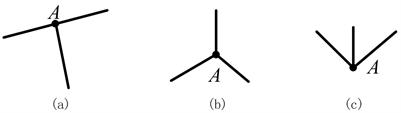Figure 2. The degree of node A is 3, but it cannot be regarded as matching

3. 变化检测Table 2. Basic change form of road object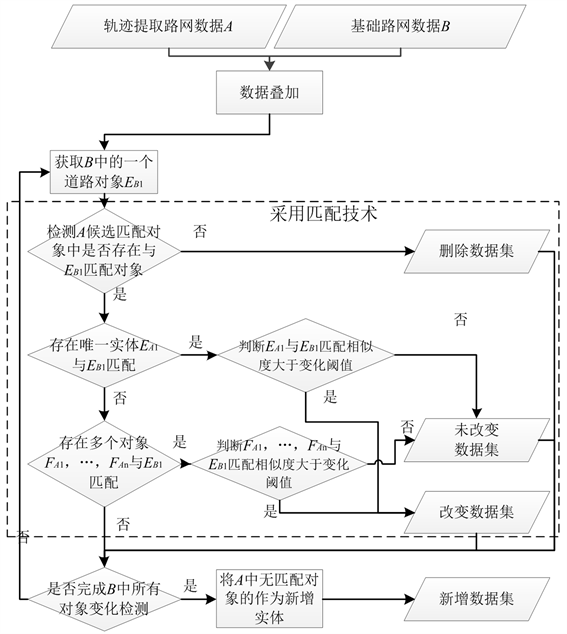Figure 3. Road network change detection flow chart

4. 实验分析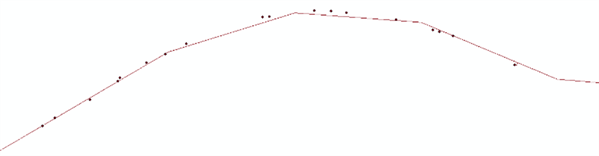Figure 4. Coordinate point of experimental areaFigure 5. Track car route

1) 长度

2) 面积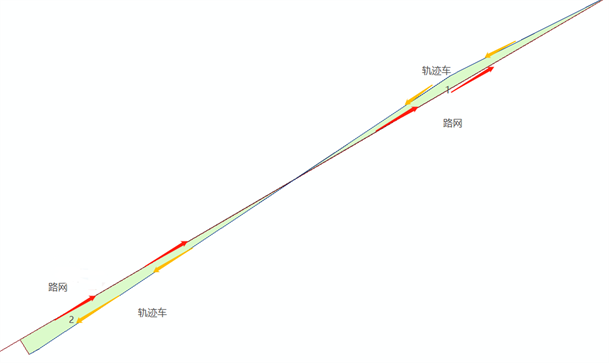Figure 6. Area matching diagram

3) 方向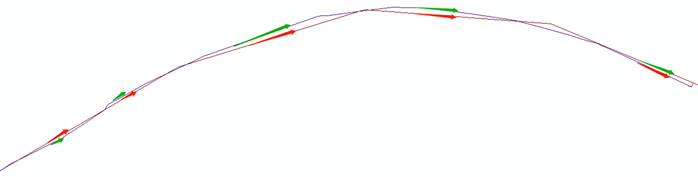Figure 7. Direction matching graph

5. 小结

NOTES

*通讯作者。

  李宇光. 海量低频浮动车数据道路匹配及行程时间估算[D]: [博士学位论文]. 武汉: 武汉大学, 2013.  任建平. 利用高分辨率遥感影像提取城市路网信息[D]: [硕士学位论文]. 兰州: 兰州大学, 2018.  闫菁. 基于高分遥感影像的道路信息快速提取技术研究[D]: [硕士学位论文]. 南昌: 江西农业大学, 2016.  张峰飞. 基于浮动车的交通信息服务系统关键技术研究[D]: [硕士学位论文]. 北京: 清华大学, 2014.  Cao, C. and Sun, Y. (2014) Automatic Road Centerline Extraction from Imagery Using Road GPS Data. Remote Sensing, 6, 9014-9033. https://doi.org/10.3390/rs6099014  Shi, C.Y., Chen, B.Y. and Li, Q.Q. (2017) Estimation of Travel Time Distributions in Urban Road Networks Using Low-Frequency Floating Car Data. ISPRS International Journal of Geo-Information, 6, 253. https://doi.org/10.3390/ijgi6080253  Liu, X.L., Kang, L., Li, M., et al. (2017) A ST-CRF Map-Matching Method for Low-Frequency Floating Car Data. IEEE Transactions on Intelligent Transportation Systems, 18, 1241-1254.https://doi.org/10.1109/TITS.2016.2604484  Zou, Y.H., Feng, Y.H., Huang, J.C., et al. (2018) Short-Term Forecast for Average Speed of Road Section Based on Floating Car Data with Support Vector Regression. Journal of Physics Conference Series, 1061, Article ID: 012017. https://doi.org/10.1088/1742-6596/1061/1/012017  楚潇蓉, 逯跃锋, 陈坤, 陆黎娟, 范俊甫. 基于支持向量机与尺度不变特征转换算法相结合的线状矢量数据匹配方法[J]. 科学技术与工程, 2019, 19(28): 35-41.  安晓亚, 杨云, 刘平芝. 基于拓扑谓词的空间拓扑关系相似性度量模型与应用[J]. 地球信息科学学报, 2013, 15(2): 159-165.